Transform, categorize, and do math with your data.

Calculated fields let you create new metrics and dimensions derived from your data. Calculated fields let you extend and transform the information flowing from your data sources and see the results in reports.

How calculated fields work

A calculated field is a formula that performs some action on one or more other fields in your data source. Calculated fields can perform arithmetic and math, manipulate text, date, and geographic information, and use branching logic to evaluate your data and return different results. The output of a calculated field can then be displayed for every row of data in charts that include that field. How this new data is displayed depends on how it's used.

For example, say you create a calculated field called Total that multiplies a quantity sold field (Qty Sold) by a unit price field (Price):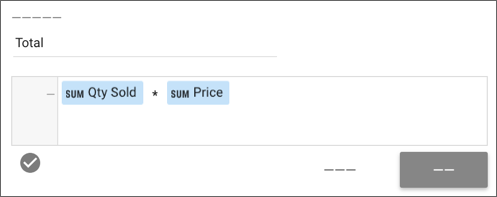When used in a table, the calculated Total field shows the product of that multiplication for each row.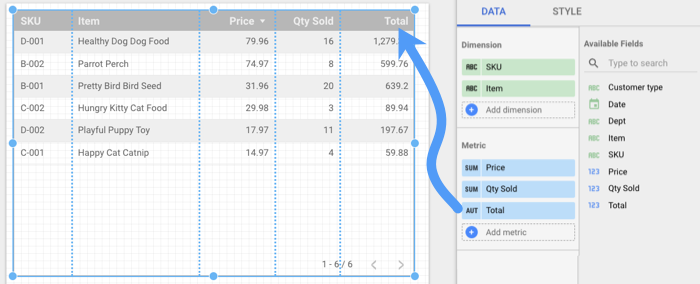When used in scorecard, the Total field displays the sum of the products for all rows in which quantity is multiplied by price.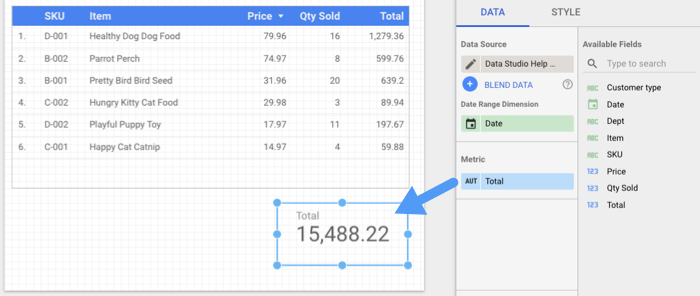Data source vs. chart-specific calculated fields

There are 2 kinds of calculated fields, determined by where you create them: in the data source, or in specific charts in a report. Each kind of calculated field offers certain advantages over the other.

Calculated fields in data sources

When you create a calculated field in a data source:

• The calculated field is available in any report that uses that data source.
• You can use a data source calculated field in charts, controls, and other calculated fields, just like a regular field.
• You can filter on a data source calculated field, just like a regular field. For example, you could set a filter property to include only items with a combined value of \$500 or more: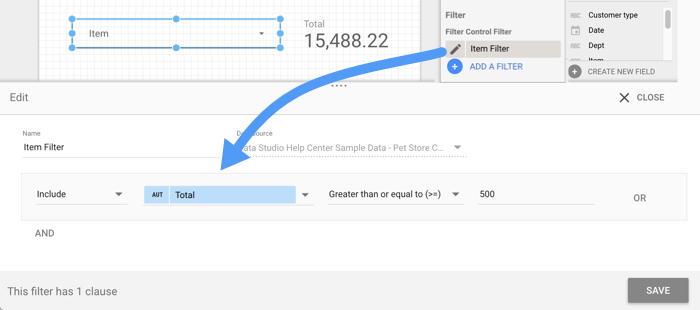Limits of data source calculated fields

Calculated fields in data sources have the following limitations:

• You can't use a data source calculated field with blended data.
• You must have edit rights to the data source to create or edit calculated fields there.

Chart-specific calculated fields

You can add calculated fields directly to a chart in your report. These chart-specific (also known as "chart-level") calculated fields can do math, use functions, and return results based CASE statements, just like calculated fields in a data source.

Chart-specific calculated fields offer some advantages over data source calculated fields:

• You can create chart-specific calculated fields based on blended data.
• You can include data source calculated fields in chart-specific calculated fields.

Limits of chart-specific calculated fields

• Chart-specific calculated fields only exist in the chart in which you create them. Creating a field in the chart does not also create it in the chart's data source.
• You can't reference other chart-specific calculated fields in your formula, even if those fields are defined in the same chart. (If you need to reference other calculated fields, use a data source calculated field.)
• To be able to create chart-specific calculated fields, you must be an editor of the report.
• Field Editing in Reports must be enabled in the data source.

The following table summarizes the differences between these 2 kinds of calculated fields.

Feature Data source calculated fields Chart-specific calculated fields
Who can create? Data source editors Report editors
Works on blended data? No Yes
Include other calculated fields? Yes No
Where can the field be used? Any report based on the data source Only the specific chart in which it was created

Data type

Just like regular fields, calculated fields have a specific semantic data type. For example, if your calculated field uses arithmetic or aggregation functions, the calculated field's data type is Number. If the calculated field uses a text function, the data type is Text.

Most date functions return a Number, except for the `TODATE` function, which returns a Date type.

You can change the data type of your calculated fields using the Type drop-down menu in the data source editor.

Aggregation

A field's aggregation determines how it can be used and summarized in your reports. If the result of a calculated field's formula is an aggregated number of some kind, the new field is a metric. If the end result is a string (text), a date, or an unaggregated number, the new field is a dimension.

Data Studio tries to assign the most appropriate aggregation to a calculated field. For most arithmetic and aggregation functions, the field aggregation is `Auto`.

You can't change the `Auto` aggregation type.

For other functions, you can specify the aggregation type using the Aggregation drop-down menu.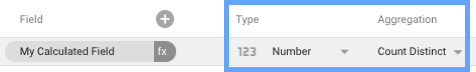What you can do with calculated fields

Calculated fields let you do the following kinds of things.

Do basic math with numeric fields

You can do simple arithmetic calculations using the normal operators:

• Subtraction: -
• Division: /
• Multiplication: *

You can construct a calculated field using any of the operators above in combination with static numeric values and unaggregated numeric fields from your data source. Use parentheses to force calculation order.

Examples

`Users / New Users`

`(Price * Quantity) * .085`

Functions let you aggregate your data in different ways, apply mathematical and statistical operations, manipulate text, and work with date and geographic information.

Examples

`SUM(Quantity)` - adds the values in the Quantity field.

`PERCENTILE(Users per day, 50)` -- returns the 50th percentile of all values of the Users per day field.

`ROUND(Revenue Per User, 0)` -- rounds the Revenue per User field to 0 places.

`SUBSTR(Campaign, 1, 5)` -- returns the first 5 characters of the Campaign field.

`REGEXP_EXTRACT(Pipe delimited values, '^([a-zA-Z_]*)(\\|)')` -- extracts the first value in a pipe delimited string.

Note the use of the double backslash to escape the | character.

`DATE_DIFF(Start Date, End Date)` -- calculates the number of days between Start Date and End Date.

`TODATE(concat(Year, '-', Month Number, '-', Day Number), "DEFAULT_DASH", "%Y%m%d")` -- create a date from concatenating separate fields containing valid date parts.

`TOCITY(Criteria ID, "CRITERIA_ID")` -- Display the associated city name from a valid Google Ads Geographical Targeting criteria ID.

Use branching logic in calculated fields

CASE statements let you perform branching "if/then/else" style logic in your calculated fields. For example, the following CASE formula categorizes the specified countries into regions, while grouping unspecified ones into an "Other" category:

``````CASE
WHEN Country IN ("USA","Canada","Mexico") THEN "North America"
WHEN Country IN ("England","France") THEN "Europe"
ELSE "Other"
END```
```